# Artificial intelligence, how does it work?

Share :
Published the 9/15/2021

Artificial intelligence can raise concerns and questions about its power over our lives and freedoms. It also raises a lot of questions among experts in the field, and also from a political point of view, with the European Union’s desire to legislate and regulate its use. To better understand AI, it is better to know how it works…

Geoffrey Hinton, Turing Award in computer science (the equivalent of the Nobel Prize in the field), known as one of the "founding fathers" of AI once asked this question: «Suppose you have a cancer that needs to be operated on and, given a choice between an «artificial surgeon», a «black box» that can’t explain how it works, but has 90% success rate, and a human who has an 80% success rate, do you want the «artificial surgeon» to be illegal?»

This was obviously deliberately provocative, but it illustrates the current understanding associated with AI. The two possibilities are presented as being in opposition, losing sight of the crucial point about artificial intelligence: humans and AI are not in opposition. AI is a tool at the disposal of humans. And humans and computers have complementary abilities: humans are good at generalizing knowledge, while AI is good at repeating. We will see why by presenting how AI works.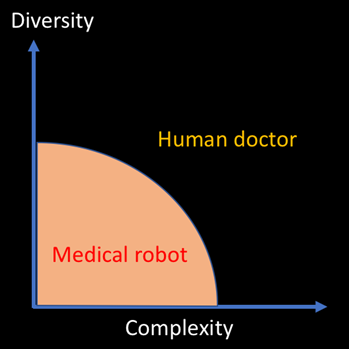Figure 1 - Humans are better at solving diverse and complex problems. Machines are very good at repetitive, low complexity problems.

Source: https://medium.com/@rbaeza_yates/human-or-robot-cf6777d15de8

A short deciphering of AI

It is important to understand the terminology associated with artificial intelligence and also to understand why AI is currently booming. Artificial intelligence is a generic term for the discipline, while the techniques used are for example, machine learning and deep learning. In order to understand how learning algorithms work, let's compare this concept with classical computer science.

Classical computer science follows the following approach: the goal is to enumerate all the possibilities in an algorithm and thus solve the problem. In Machine Learning,the aim is to make a "simple" algorithm understand how to solve a complex problem (for which it is impossible to enumerate all the possibilities) using data from the problem.

Finally, Deep Learning consists of making a (slightly less) "simple" algorithm, called neural networks, understand how to solve an (even more) complex problem, thanks to (even more) data taken from this problem. Deep Learning is a type of Machine Learning algorithm.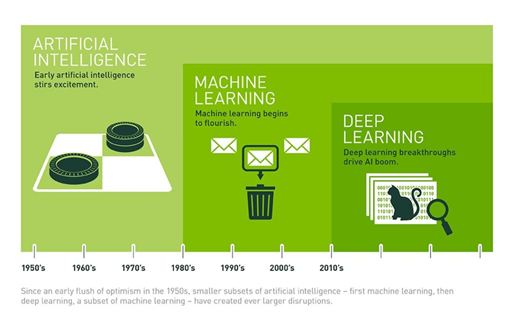Figure 2 - A history of artificial intelligence, also illustrating the interweaving of techniques.

What do we mean when we talk about AI?

Well, Deep Learning has been the talk of the town in recent years. It is a relatively old concept that went through a great period of dearth between the 1980s and 2010. However, these techniques are currently experiencing a boom thanks to Big Data and to the computational capacities that have been greatly developed. As a result, these data-intensive algorithms can now be sufficiently "fed" with data to be effective, which was not the case in the past. Advances in computational capacity mean that training can be done in a reasonable amount of time.

But what is Deep Learning? It is a category of algorithm inspired by the functioning of the brain. It is a network of so-called neurons, which are interconnected and used to perform mathematical operations. Thus, Deep Learning algorithms can be visualised as successive layers of neurons, each layer using the results of the previous layers' calculations to perform new calculations.Figure 3 - How a neural network works

Deep Learning is particularly successful for several reasons: these techniques can produce any type of data (image, text, sound, number...) unlike Machine Learning. These algorithms can therefore calculate and solve more complex problems. Finally, the preliminary work of formatting and analysing the data is generally less complex with Deep Learning algorithms.

A story of learning

As you have now understood, for learning algorithms to learn, they need to be provided with data for training. This is true for any type of learning algorithm, be it Machine Learning or Deep Learning.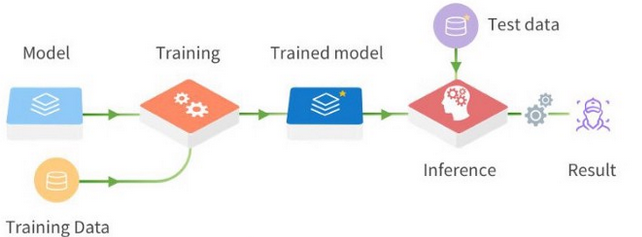Figure 4 - A classic Deep Learning process. A model, the Deep Learning algorithm, will receive training data as input. Once the model is trained, it is applied to unknown data to test it and then in the environment in which it will be used.

Several types of training exist, and the two most common are supervised learning and unsupervised learning. The first one consists of providing labelled data to the model so that it understands the features associated with each of these labels. Suppose we are working on creating a classification model of animal images. To train the model, the model will be given a large number of animal images with an associated label corresponding to the animal that is present in the image. The algorithm will then understand, thanks to its training, which characteristics are specific to the images of each animal breed.

In the case of unsupervised learning, we will this time provide a large amount of data to the model, but without specifying a label. Thus, it will have to figure out on its own how to distinguish the categories, but also to define itself what these categories are. It is still noteworthy that for each of these techniques, we never explain to the algorithms the characteristics of the elements they have to analyse. That's the whole point: it will understand what these characteristics are on its own.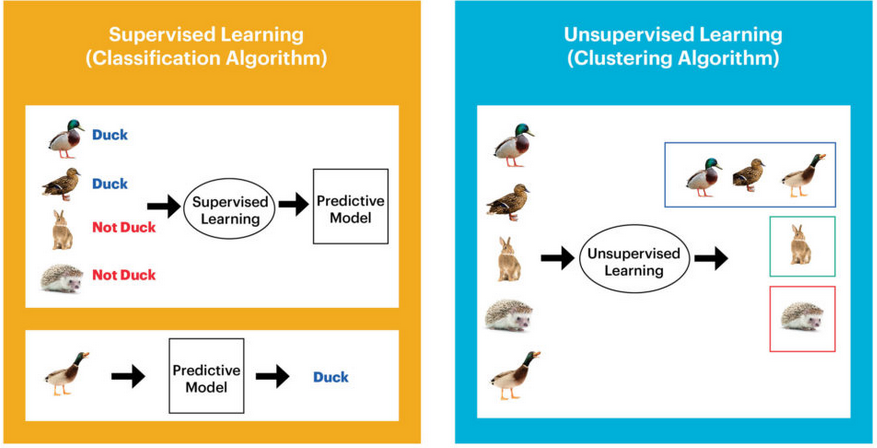Figure 5 - Illustration of the different types of learning. On the left, supervised learning illustrated by classification. This process consists in associating a class or label to an input data. The unsupervised process, here being a clustering algorithm, i.e. data partitioning, consists in grouping similar data into clusters. These groups, unlike the classification process, are not specified in the training data.

How it works in practice

Now that we understand the principle of training, let's look at what is going on from a mathematical point of view. In order for the problem to be solved by a machine learning algorithm, two major assumptions must be verified. Assumption 1, the problem follows an unknown mathematical function (or rather distribution), which the model must approximate. This assumption is mainly used to ensure that the process we are trying to model is not completely random.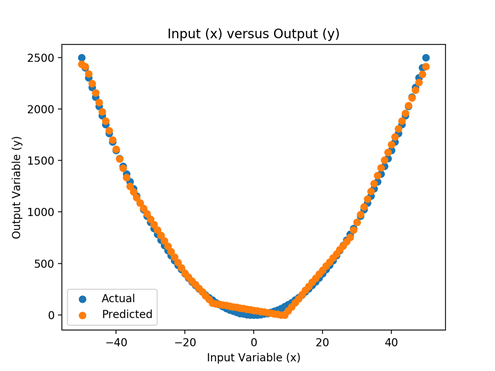Figure 6 - Simplification of the operation of a trained learning algorithm. In blue, the unknown function that must be approximated. In orange, the function that the algorithm will have successfully learned using the training data.

Assumption 2, the training data correctly describes the "unknown function". This second assumption is particularly important. Indeed, a learning algorithm, no matter how good it may be, is totally dependent on the input data. If we train an algorithm to classify animal images, the trained algorithm will probably perform well for the categories of animals it has already seen. However, it will have infinite difficulty in generalising the features of an animal to an animal species it has never seen. Similarly, he will be extremely dependent on the representativeness of the images: if the animals are always in front, he will probably have difficulty categorising animals from the back. Similarly, if he is trained only to recognise Bernese Mountain dogs, he will probably have difficulty understanding what a Yorkshire is.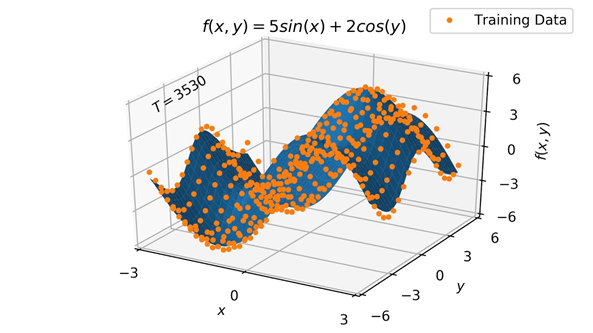Figure 7 - Illustration of the need for training data representative of the function being sought. In blue, the unknown function. In orange, the data supposed to represent this function and allow the model to "guess" it.

Artificial intelligence algorithms are now revolutionising many fields but still have limitations. Currently, AI only approximates a function describing the data it has been trained on. It responds to a very specific problem and has little ability to learn what is not in the training data. Most importantly, the AI has not learned how to learn, it has simply learned a mathematical function that approximates the underlying "unknown function" that is supposed to be described by the training data. So we are still far from science fiction cases of omniscient AI and even human intelligence.

Sources :

 https://medium.com/@rbaeza_yates/human-or-robot-cf6777d15de8

 https://blog.westerndigital.com/machine-learning-pipeline-object-storage/

Tags :
Share :